Courses

Chemical Kinetics

20 Questions MCQ Test GATE Chemistry Mock Test Series | Chemical Kinetics

Description
Attempt Chemical Kinetics | 20 questions in 60 minutes | Mock test for Chemistry preparation | Free important questions MCQ to study GATE Chemistry Mock Test Series for Chemistry Exam | Download free PDF with solutions
QUESTION: 1

If A reacts to form either B or C according to reactions,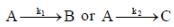if E1 is the activation energy for the first reaction and E2 is the activation energy for the second reaction then Ea, the observed activation energy for the disappearance of A is

Solution: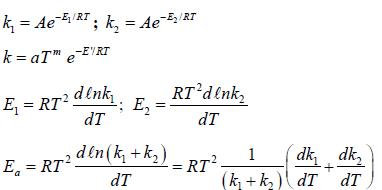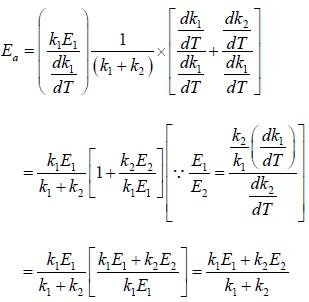QUESTION: 2

The following reaction was carried out in water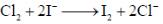the initial concentration of was 0.25 molL–1 and the concentration after 10 min was 0.23 molL–1. The rate of disappears of I– (in untis of M min–1) and rate of appearance of I2​.

Solution: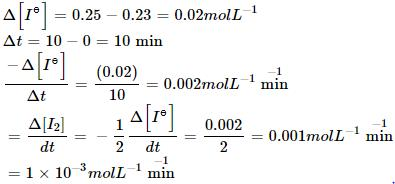QUESTION: 3

For the reaction, A + B → C +D, Δh = 20 kJ/mole. The activation energy of the forward reaction is 85 kJ/mole. The activation energy of the reverse reaction

Solution: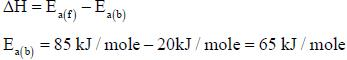*Answer can only contain numeric values
QUESTION: 4

Consider the following variation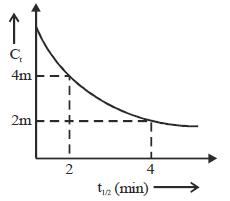The order of reaction is _______________

Solution:

For second order when concentration halved t1/2 become double.

*Answer can only contain numeric values
QUESTION: 5

For the following sequential reaction,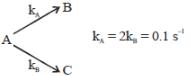time at which [B] maximum is _____(in sec).

Solution: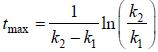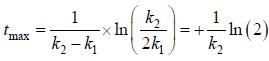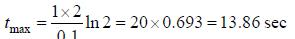*Answer can only contain numeric values
QUESTION: 6

The percent transmittance of a solution having absorbance (optical density) 1.0 is ____________

Solution: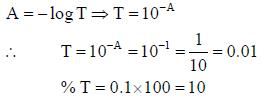QUESTION: 7

Which of the following curves represent(s) a zero order reaction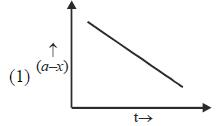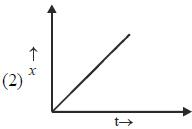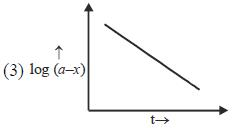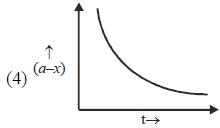Solution:
QUESTION: 8

A catalyst increases the rate of a chemical reaction by
(1) increasing the activation energy
(2) decreasing the activation energy
(3) increasing the average K.E. of the molecule
(4) increasing the number of active molecules.

Solution:
QUESTION: 9

The rate of the elementary reaction at 25oC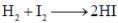Is given by rate = 1.7 x 10-18[H2][I2] - 2.4 x 10-21 [HI]2
The rate of decomposition of gaeseous HI to H2 and I2 at 25ºC is given by the equilibrium constant for the formation of HI from H2 and I2 at 25ºC is

Solution: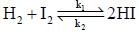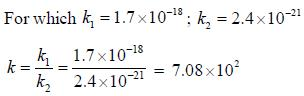QUESTION: 10

Time constant τ is the required for the conc. of a reactant to fall to 1/e of its initial value then?

Solution: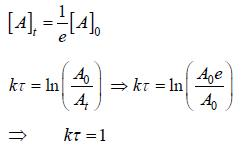QUESTION: 11

For the first order reaction,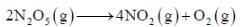A is 4.3 x 1013 s-1 and Ea is 103.35 KJ/mole. The value of k at 300K is

Solution: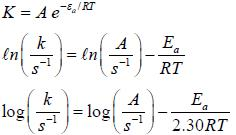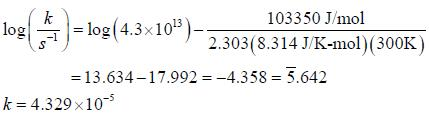QUESTION: 12

The frequency factors for a unimolecular gas reaction occuring at 473K is 2.5×1013 s–1 . The value of entropy of activation is

Solution: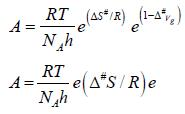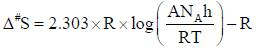QUESTION: 13

Cyclohexane inter converts between a chair and a boat structure. The activation parameters for the reaction from the chair to boat form of the molecule are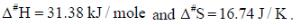The value of standard Gibbs energy of activation and the rate constant and the rate constant for this reaction at 325K

Solution: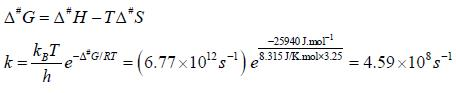QUESTION: 14

The rate law for the reaction between Co(g) to form phosgene (Cl2CO)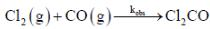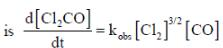Show that the following mechanism is consistent with this rate law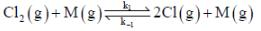(Fast equilibrium)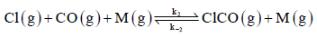(Fast equilibrium)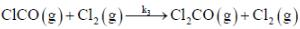(Slow)
Where M is any gas molecule present in the reaction container. The value of kobs in terms of the rate constants for the individual. Steps of the reaction mechanism

Solution: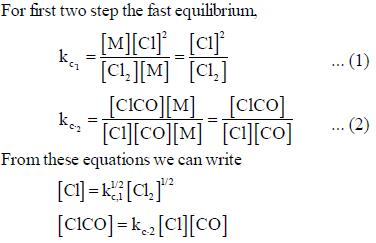Using the third step of the reaction mechanism to write d[CL2CO] / dt and equations  (1) and (2)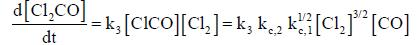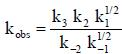*Answer can only contain numeric values
QUESTION: 15

A substance, when dissolved in water at 10-3 M, absorbs 10% of incident radiation in a path of 1 cm length. What should be the concentration of the solution if it were to absorb 90% of the same radiation?

Solution: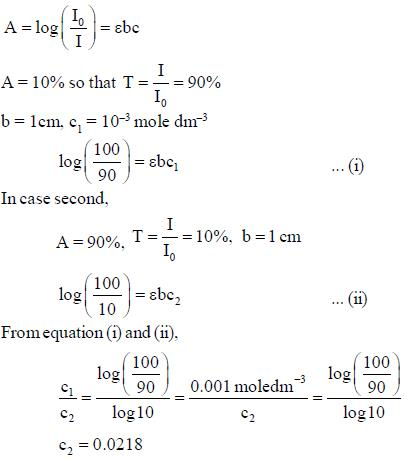*Answer can only contain numeric values
QUESTION: 16

For a reaction with rate equation - dc/dt = kc2, Co and C are the concentration of the reactant at time zero and t respectively. If 10 minutes were required for C0 to becomes C0/2 the time required for Co to becomes C0/4 is ____ min. (Answer should be an integer).

Solution: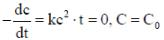On integration by taking time 0 to t, we get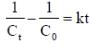...(i)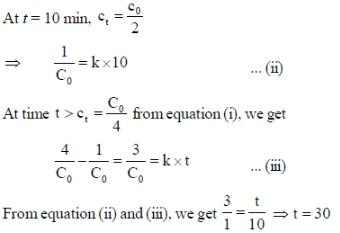*Answer can only contain numeric values
QUESTION: 17

The concentration of non-competitive inhibitor(k1 = 2.9 x 10-4 moldm-3) needed to yield to 90% inhibiten of an enzyme catalysed reaction is ______mol dm-3. (Round off to two decimal places).

Solution: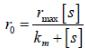...(i)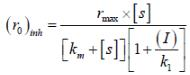...(ii)
(i)/(ii), we get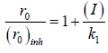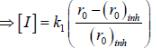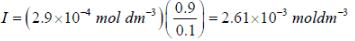*Answer can only contain numeric values
QUESTION: 18

During Nuclear-explosion, one of the product is 90Sr with half-life is 28.1 year. If 1μg od 90Sr was absorbed in the bones of a newly born baby instead of calcium. How much of it will remain after 10 year if it is not lost metabolically? (in unit of μg) (Round off to two decimal places).

Solution: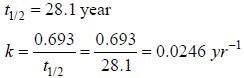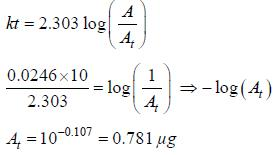*Answer can only contain numeric values
QUESTION: 19

In a homogeneous catalytic reaction, 1.0 M of a substrate band, 1.0 μM of a catalyst yield 1.0 mM of a product in 10S. The turnover frequency (TOF) of the reaction(s-1) is ______(answer should be an integer)

Solution: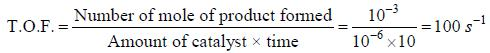QUESTION: 20

In the Linewear Burk plot of (initial rate)–1 vs (initial substrate concentration)–1. For an enzyme catalyzed reacts following Michael Menten mechanism. The y-intercept is 6000 M–1s. If the initial enzyme concentration is 1×10–5 M. The turn over number is

Solution: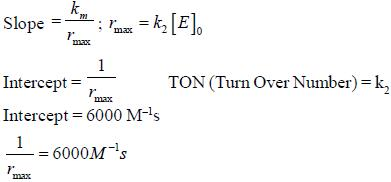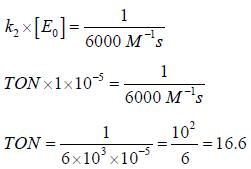Use Code STAYHOME200 and get INR 200 additional OFF Use Coupon Code

Track your progress, build streaks, highlight & save important lessons and more!

Similar ContentRelated tests